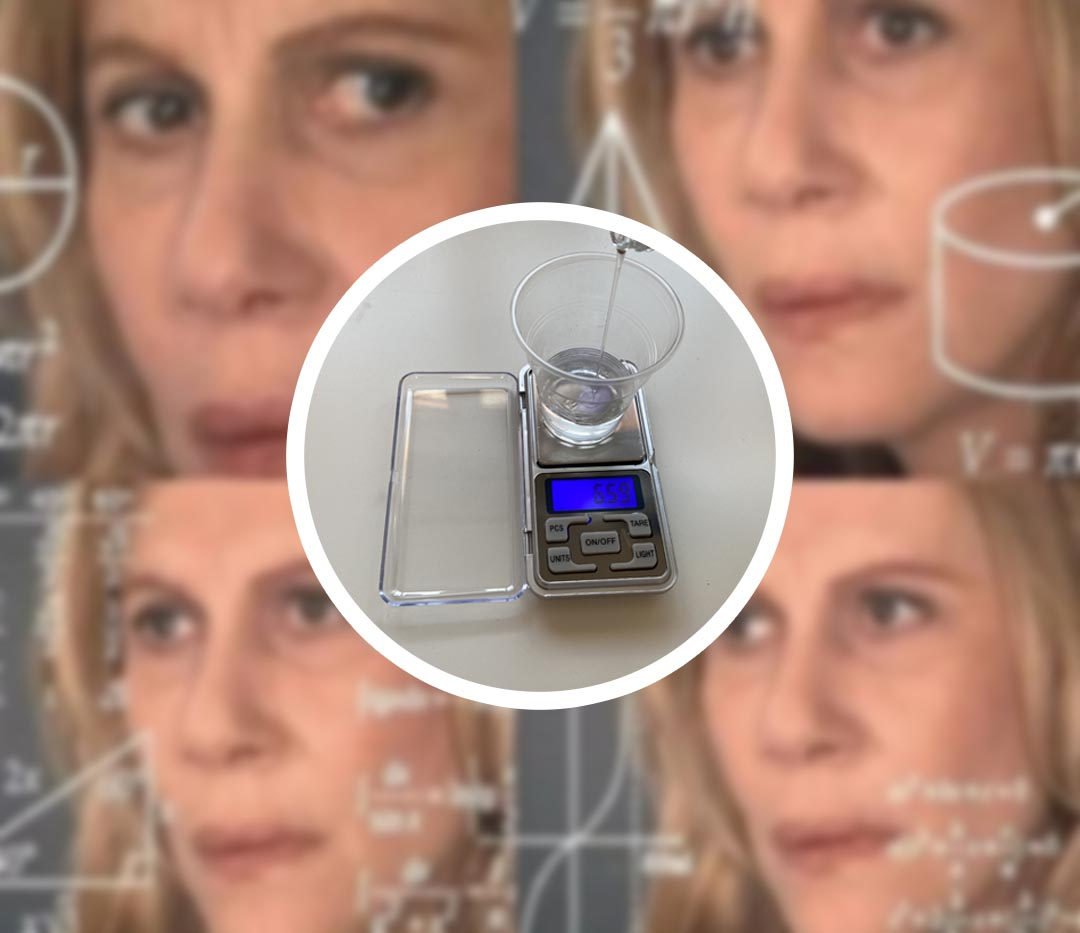Cart

#### Technical help and shipping

07596 850968# HOW TO CALCULATE THE QUANTITY OF A AND B IN EPOXY RESINS

One of the most frequently encountered difficulties when it comes to Epoxy Resin is the accuracy needed in calculating the proportions between the two components. Although the mathematical proportion is somewhat elementary, it is very easy to fall into confusion and get the relationship wrong, risking compromising the final result.
Here is a small guide to correctly calculate the ratio between A and B.

#### 1 – THE EMPLOYMENT RATIO

Don’t be frightened by this term! The use ratio is simply the ratio that defines the quantity of product A and product B needed to catalyze the product well. Being two-component it is essential that the Resin and Hardener are dosed with extreme precision. To simplify things, we can therefore define the use ratio as the amount of A and B to be used to harden our compound.

#### 2 – CALCULATE THE EMPLOYMENT RATIO GIVEN A CERTAIN QUANTITY OF A

It is very likely that, especially the first few times, you will be required to do some small tests to understand how the resin works and whether the purchased product is really for you. When you do small experiments or small artifacts, you may not already have an established quantity of resin but you proceed in a fairly random way. How then do you calculate the quantity of B to be mixed with x quantity of A?

As you can see, on the label of each product there is a mathematical proportion: 100: X (where X corresponds to the amount of hardener that changes depending on the type of product).
100” indicates instead the universal quantity of Resin A that you will choose to use, therefore at 100 (grams, kilos etc.) of A, it will be necessary to mix X (grams, kilos, etc.) of B.

Ex. : Transparent Epoxy Resin, 100: 60
Epoxy resin Epoxy5-five, 100: 55

If your resin has a ratio of 100: 55, it will mean that, for example, to 100 grams of A, 66 grams of B or, more simply, 55% will be added.
So far, nothing simpler but how to calculate the quantity of B when A does not correspond to 100?
Also in this case, we will have to rely on a very simple mathematical operation that will serve to find the coefficient with which you will multiply the quantity of A.

A (Resin) = 100; B (Hardener) = 66
66/100 = 0,66 – COEFFICIENT OF CALCULATION
By multiplying any amount of A by this coefficient, you will get the grams of B to add to your compound.

Clearly, by changing the employment ratio, the coefficient will also change

Ex: Ratio A 100: B 55; Coefficient = 55/100 = 0.55
Ratio A 100: B 60; Coefficient = 60/100 = 0.60

Now let’s see a more concrete example:
I poured 32 g of resin (ratio 100: 66), how many grams of B should I add?

32 g of A X 0.66 = 21.12 g of B.

To calculate the quantity of B, it will therefore only be necessary to multiply the grams of A by the figure of the ratio of use positioned on the right, preceded by a 0.

#### 3 – CALCULATE THE USE RATIO GIVEN A DETERMINED TOTAL QUANTITY OF RESIN

If you choose to continue your adventure in the world of resin, it will certainly happen that, after having made a certain calculation, you will have obtained the total amount of hardest resin to use.
This time you will not start from a certain quantity of A but, on the contrary, from the total quantity that you will have to go to “separate” to respect the correct employment ratio.
If you need to use, for example, a total of 58.40 kg of a compound with an application ratio of 100: 66, here is the calculation you will have to do initially:

Total 58.40 kg / 1.66 = A. A = 35.18 Kg.

To calculate A, given a total quantity of compound, it will therefore only be necessary to divide the total quantity by the figure of the use ratio positioned on the right, preceded by a 1.
At this point, to calculate the amount of B, you just have to perform the operation already listed in point 2.

CALCULATION EXAMPLES FOR SOME TYPES OF RESIN PRO RESINS STARTING FROM A TOTAL QUANTITY OF COMPOUND (A + B) EQUAL TO 50 KG.

Transparent resin cast up to 2 cm – RATIO 100: 60
50 Kg / 1.60 = 31.25 Kg of A
31.25 Kg (A) X 0.60 = 18.75 Kg of B

Epoxy5-five resin cast up to 5 cm – RATIO 100: 50
50 Kg / 1.50 = 33.33 Kg of A
33.33 Kg (A) X 0.50 = 16.66 Kg of B

Art Pro resin – RATIO 100: 66
50 Kg / 1.66 = 30.12 Kg of A
30.12 Kg (A) X 0.66 = 19.87 Kg of B

Art Pro Deluxe resin – RATIO 100: 70
50 Kg / 1.70 = 29.41 Kg of A
29.41 Kg (A) X 0.70 = 20.58 Kg of B

EpoxyFood resin – RATIO 100: 55
50 Kg / 1.55 = 32.25 Kg of A
32.25 Kg (A) X 0.55 = 17.74 Kg of B

Top Pro Heat Resistant resin – RATIO 100: 80
50 Kg / 1.80 = 27.77 Kg of A
27.77 Kg (A) X 0.80 = 22.22 Kg of B

Epoxy Table resin casts up to 10 cm – RATIO 100: 25
50 Kg / 1.25 = 40 Kg of A
40 Kg (A) X 0.25 = 10 Kg of B

Fast support

Our staff is always available

100% Secure Checkout

PayPal / MasterCard / Visa

Fast shipping

Fast Shipping!